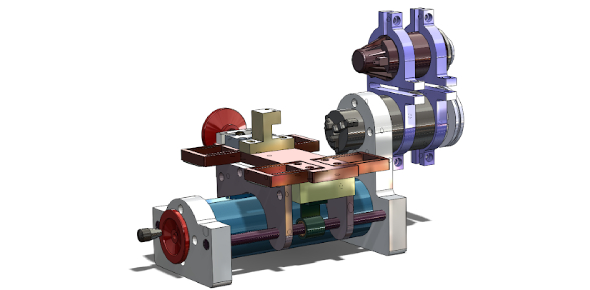# Elements Of Mechanical Engineering Quiz!

20 Questions | Attempts: 605
ShareSettings.

• 1.
Forces are called concurrent when their lines of action meet in:
• A.

One point

• B.

Two points

• C.

Plane

• D.

Perpendicular planes

• 2.
A force acting on a body may:
• A.

introduce internal stresses

• B.

Balance the other forces acting on it

• C.

Retard its motion

• D.

All the above

• 3.
Effect of a force on a body depends upon:
• A.

Magnitude

• B.

Direction

• C.

Position or line of action

• D.

All of the above

• 4.
If a number of forces act simultaneously on a particle, it is possible:
• A.

Not a replace them by a single force

• B.

to replace them by a single force

• C.

to replace them by a single force through C.G.

• D.

To replace them by a couple

• 5.
The unit of work or energy in S.I. units.
• A.

Newton

• B.

pascal

• C.

Kilogram meter

• D.

Joule.

• 6.
A force is completely defined when we specify:
• A.

Magnitude

• B.

direction

• C.

Point of application

• D.

all of the above

• 7.
Which is the correct statement about the law of polygon of forces?
• A.

If any number of forces acting at a point can be represented in direction and magnitude by the sides of a polygon taken in order, then the forces are in equilibrium

• B.

if any number of forces acting at a point can be represented by the sides of a polygon taken in order, then the forces are in equilibrium

• C.

if any number of forces acting at a point can be represented in direction and magnitude by the sides of a polygon, then the forces are in equilibrium

• D.

if a polygon representing forces acting at a point is closed then forces are in equilibrium

• 8.
Forces are called coplanar when all of them acting on body lie in:
• A.

perpendicular planes

• B.

One plane

• C.

One point

• D.

Similar planes

• 9.
The unit of force in S.I. units is:
• A.

kilogram

• B.

Newton

• C.

Watt

• D.

Dyne

• 10.
Lami's theorem is applicable for:
• A.

3 forces in any plane

• B.

3 forces at different plane

• C.

3 forces which are coplanar

• D.

3 forces which are concurrent and coplanar

• 11.
The algebraic sum of the resolved parts of a number of forces in a given direction is equal to the resolved part of their resultant in the same direction. This is as per the principle of:
• A.

Resolution of forces

• B.

Balance of force

• C.

Dependence of forces

• D.

Independence of forces

• 12.
According to law of triangle of forces:
• A.

If three forces acting upon a particle are represented in magnitude and direction by the sides of a triangle, taken in order, they will be in equilibrium

• B.

Three forces acting at a point can be represented by a triangle, each side being proportional to force

• C.

If three forces acting at a point are in equilibrium, each force is proportional to the sine of the angle between the other two

• D.

three forces acting at a point will be in equilibrium

• 13.
If two forces of magnitude P and 2P act at an angle 60°, their resultant will be:
• A.

2.65P

• B.

3P

• C.

2.59P

• D.

2.44P

• 14.
Which of the following is not a scalar quantity?
• A.

Acceleration

• B.

Density

• C.

Time

• D.

Mass

• 15.
The weight of a body is due to:
• A.

Gravitational force of attraction towards the center of the earth

• B.

Force of attraction experienced by particles

• C.

Gravitational pull exerted by the earth

• D.

Forces experienced by body in atmosphere

• 16.
The figure shows a particular position of 200 mm connecting rod AB and 80 mm long crank BC. At this position, the connecting rod of the engine experience a force of 3000 N on the crank pin at B. Find its horizontal component.
• A.

2814.2 N

• B.

1039.2 N

• C.

507.1 N

• D.

568 N

• 17.
The figure shows a particular position of 200 mm connecting rod AB and 80 mm long crank BC. At this position, the connecting rod of the engine experience a force of 3000 N on the crank pin at B. Find its vertical component.
• A.

2814.2 N

• B.

1039.2 N

• C.

507.1 N

• D.

568 N

• 18.
Determine the tension in cables AB necessary to support the 60-kg cylinder in Figure.
• A.

420 N

• B.

450 N

• C.

457.6 N

• D.

475.6 N

• 19.
Determine the tension in cables BC necessary to support the 60-kg cylinder in Figure.
• A.

420 N

• B.

450 N

• C.

476

• D.

457 N

• 20.
Determine the magnitude of the component force F in the given figure.
• A.

245 lb

• B.

273 lb

• C.

500 lb

• D.

Can't determined

## Related TopicsBack to top
×

Wait!
Here's an interesting quiz for you.